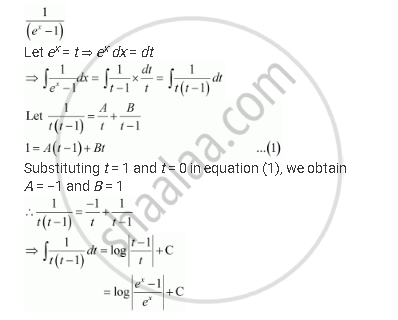Share

# Integrate the Rational Functions 1/(E^X -1)[Hint: Put Ex = T] - CBSE (Commerce) Class 12 - Mathematics

ConceptMethods of Integration - Integration Using Partial Fractions

#### Question

Integrate the rational functions 1/(e^x -1)[Hint: Put ex = t]

#### SolutionIs there an error in this question or solution?

#### Video TutorialsVIEW ALL 

Solution Integrate the Rational Functions 1/(E^X -1)[Hint: Put Ex = T] Concept: Methods of Integration - Integration Using Partial Fractions.
S